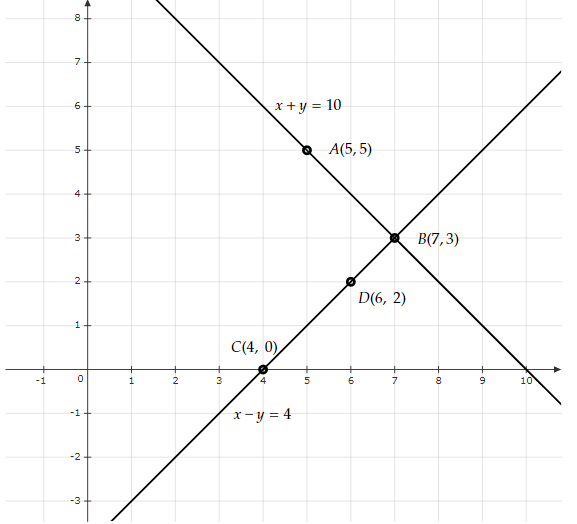# Form the pair of linear equations in the following problems, and find their solution graphically:10 students of class X took part in Mathematics quiz. If the number of girls is 4 more than the number of boys, find the number of boys and girls who took part in the quiz.

Given:

10 students of class X took part in Mathematics quiz. The number of girls is 4 more than the number of boys.

To do:

We have to form the pair of linear equations and solve them graphically. Also, we have to find the number of boys and girls who took part in the quiz.

Solution:

Let the number of girls and boys in the class be $x$ and $y$ respectively.

According to the question,

$x + y = 10$.....(i)

$x - y = 4$.....(ii)

To represent the above equations graphically we need at least two solutions for each of the equations.

For equation $x+y=10$,

$y=10-x$

If $x=5$ then $y=10-5=5$

If $x=7$ then $y=10-7=3$

 $x$ $5$ $7$ $y$ $5$ $3$

For equation $x-y=4$,

$y=x-4$

If $x=4$ then $y=4-4=0$

If $x=6$ then $y=6-4=2$

 $x$ $4$ $6$ $y$ $0$ $2$

The above situation can be plotted graphically as below:The lines AB and CD represent the equations $x+y=10$ and $x-y=4$ respectively.

The solution of the above equations is the point of intersection.

Therefore,

Number of girls $x=7$

Number of boys $y=3$

The number of boys and girls who took part in the quiz are $3$ and $7$ respectively.

Updated on: 10-Oct-2022

38 Views